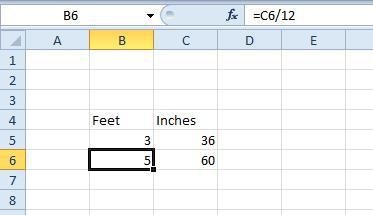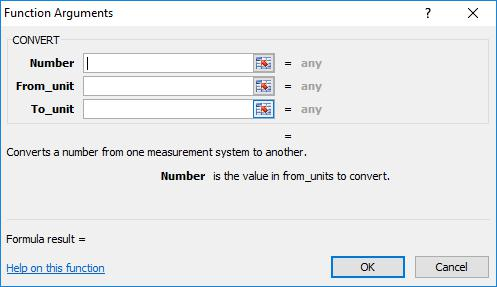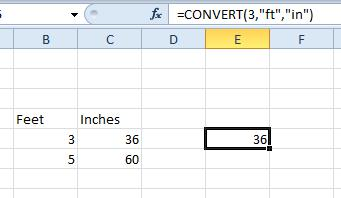# How To Convert Feet to Inches in Excel

Excel users will often need to include dimension figures in their spreadsheets. Feet and inches are two of the more regular measurement units for length, so some users will need to convert feet to inch values in their spreadsheets; or perhaps the other way around. These are a few of the ways that you can convert feet to inches, and vice versa, in Excel.### How to Convert Feet to Inch Values without a Function

You don’t always need to add a function to convert feet values to inches in Excel spreadsheets. Instead, you can manually add a formula to the fx bar. There are 12 inches in a foot, so you multiply the value by 12 to convert feet to inches. Alternatively, you divide the inch value by 12 to convert it to feet.

Open a blank Excel spreadsheet and enter ‘Feet’ in cell B4 and ‘Inches’ in C4, which are two column headings. Enter ‘3’ in cell B5, which is the length measurement in feet. Next, you should select cell C5 and click inside the fx function bar. Now input ‘=B5*12’ in the function bar and press the Enter key. Cell C5 should return the value 36 inches as shown in the snapshot below.To convert inches to feet, select cell B6 and then click inside the fx bar. Input ‘=C6/12′ in the fx bar and press the Enter key. Now input the number of inches in cell C6. For example, if you enter ’60’ cell B6 will return a value of five feet as shown directly below.### Converting Feet to Inches with CONVERT

Excel has a CONVERT function that enables you to convert one measurement unit to another. With that function you can convert distance, mass, time, temperature, volume, area, prefix and energy units. The syntax for the CONVERT function is: CONVERT(number, from_unit, to_unit). Number is the overall value to convert from one unit to another, from_unit is the original unit and to_unit is the new unit to convert to.

To convert feet to inches with CONVERT, select cell E5 and press the Insert Function button. Select All from the Or select a category drop-down menu. Then you can select CONVERT on the Insert Function window, and press the OK button to open its window in the snapshot below.Now input the value ‘3’ in the Number field box. Enter “ft” into the From_unit text box and “in” (with quote marks included) in the To_unit text box. The units are also case-sensitive, so entering “FT” will return an N/A error value in the cell. Press OK to add the CONVERT function to the spreadsheet, which should return the value 36 as shown in the snapshot directly below.You can convert inches to feet by swapping the units round. So enter ’36’ in the Number box, “in” in the From_unit box and “ft” in the To_unit text field instead. Then the function will convert the value from feet to inches as below.### Convert Feet to Inches with Kutools

Kutools is a handy Excel extension that adds more than 120 new tools to the application. That also includes a unit converter which you can convert feet values to inches with. Kutools is retailing at \$39, and you can add it to the full versions of Excel 2016, ’13 and ’10. There’s also a 30-day Kutools demo for you to try out.

When you’ve added Kutools to Excel, you’ll find a Kutools tab at the top of the application’s window. First, select the cell, or range of cells, that includes the value to convert to inches. Then click the Kutools tab, Content Converter and select Unit Conversion to open the tool’s window.Click the Units drop-down menu and select Distance from there. Select Foot from the left list box. Then select Inch in the right list box. Press the OK button to convert the selected cell values to inches and close the window.

Note that the converter tool will overwrite all the original values in the cells. So if you still want to keep the original feet values as well, click the Add results as comment check box on the Unit Conversion window before pressing OK. That will show the inch values in comment boxes instead of the selected cells.

So that’s how you can convert feet to inches in Excel spreadsheets. You can also convert mile, yard, nautical mile and meter distance units with the CONVERT function much the same by entering the “mi,” “yd,” “Nmi” and “m” units.

Disclaimer: Some pages on this site may include an affiliate link. This does not effect our editorial in any way.

Todays Highlights